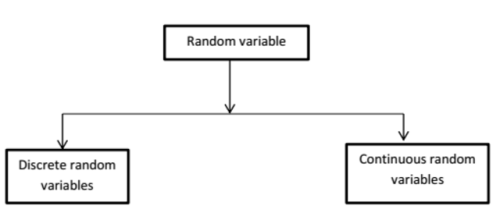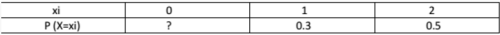Courses

# Random Variables Computer Science Engineering (CSE) Notes | EduRev

## Computer Science Engineering (CSE) : Random Variables Computer Science Engineering (CSE) Notes | EduRev

The document Random Variables Computer Science Engineering (CSE) Notes | EduRev is a part of the Computer Science Engineering (CSE) Course GATE Computer Science Engineering(CSE) 2022 Mock Test Series.
All you need of Computer Science Engineering (CSE) at this link: Computer Science Engineering (CSE)

Random Variable

Random variable is basically a function which maps from the set of sample space to set of real numbers. The purpose is to get an idea about result of a particular situation where we are given probabilities of different outcomes. See below example for more clarity.

Example :

Suppose that two coins (unbiased) are tossed

X = number of heads. [X is a random variable or function]

Here, the sample space S = {HH, HT, TH, TT}.

The output of the function will be :
X(HH) = 2
X(HT) = 1
X(TH) = 1
X(TT) = 0

Formal definition :
X: S -> R
X = random variable (It is usually denoted using capital letter)
S = set of sample space
R = set of real numbers

Suppose a random variable X takes m different values i.e. sample space X = {x1, x2, x3………xm} with probabilities P(X=xi) = pi; where 1 ≤ i ≤ m. The probabilities must satisfy the following conditions :

1. 0 <= pi <= 1; where 1 <= i <= m
2. p1 + p2 + p3 + ……. + pm = 1 Or we can say 0 ≤ pi ≤ 1 and ∑pi = 1.

Hence possible values for random variable X are 0, 1, 2.
X = {0, 1, 2} where m = 3
P(X=0) = probability that number of heads is 0 = P(TT) = 1/2*1/2 = 1⁄4.
P(X=1) = probability that number of heads is 1 = P(HT | TH) = 1/2*1/2 + 1/2*1/2 = 1⁄2.
P(X=2) = probability that number of heads is 2 = P(HH) = 1/2*1/2 = 1⁄4.

Here, you can observe that
1) 0 ≤ p1, p2, p3 ≤ 1
2) p1 + p2 + p3 = 1/4 + 2/4 + 1/4 = 1

Example :
Suppose a dice is thrown X = outcome of the dice. Here, the sample space S = {1, 2, 3, 4, 5, 6}. The output of the function will be:

1. P(X=1) = 1/6
2. P(X=2) = 1/6
3. P(X=3) = 1/6
4. P(X=4) = 1/6
5. P(X=5) = 1/6
6. P(X=6) = 1/6

See if there is any random variable then there must be some distribution associated with it.Discrete Random Variable:

A random variable X is said to be discrete if it takes on finite number of values. The probability function associated with it is said to be PMF = Probability mass function.
P(xi) = Probability that X = xi = PMF of X = pi.

1. 0 ≤ pi ≤ 1.
2. ∑pi = 1 where sum is taken over all possible values of x.

The examples given above are discrete random variables.

Example:- Let S = {0, 1, 2}Find the value of P (X=0):
Sol:- We know that sum of all probabilities is equals to 1.
==> p1 + p2 + p3 = 1
==> p1 + 0.3 + 0.5 = 1
==> p1 = 0.2

Continuous Random Variable:

A random variable X is said to be continuous if it takes on infinite number of values. The probability function associated with it is said to be PDF = Probability density function
PDF: If X is continuous random variable.
P (x < X < x + dx) = f(x)*dx.

1. 0 ≤ f(x) ≤ 1; for all x
2. ∫ f(x) dx = 1 over all values of x

Then P (X) is said to be PDF of the distribution.

Example:- Compute the value of P (1 < X < 2).

Such that f(x) = k*x^3; 0 ≤ x ≤ 3
= 0; otherwise
f(x) is a density function

Solution:- If a function f is said to be density function, then sum of all probabilities is equals to 1. Since it is a continuous random variable Integral value is 1 overall sample space s.
==> K*[x^4]/4 = 1 [Note that [x^4]/4 is integral of x^3]
==> K*[3^4 – 0^4]/4 = 1
==> K = 4/81
The value of P (1 < X < 2) = k*[X^4]/4 = 4/81 * [16-1]/4 = 15/81.

Offer running on EduRev: Apply code STAYHOME200 to get INR 200 off on our premium plan EduRev Infinity!

## GATE Computer Science Engineering(CSE) 2022 Mock Test Series

131 docs|159 tests

,

,

,

,

,

,

,

,

,

,

,

,

,

,

,

,

,

,

,

,

,

;